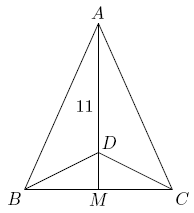# Not as They Seem to Be

Geometry Level 4Triangle $ABC$ is isosceles, with $AB=AC$ and altitude $AM=11.$ Suppose that there is a point $D$ on $\overline{AM}$ with $AD=10$ and $\angle BDC=3\angle BAC.$ Then the perimeter of $\triangle ABC$ may be written in the form $a+\sqrt{b},$ where $a$ and $b$ are integers. Find $a+b.$

×